# Tamil Nadu Board Class 12 Physics Practical

Physics experiments form the basis for Class 12 science and help to engage more students by providing them with hands-on experience doing practicals. It also helps the students to understand the concepts thoroughly and more effectively. Students can refer to these Class 12 Physics practical details of Tamil Nadu Education Board that are given and can prepare better for the exams. Students are advised to refer to the experiments and activities from the Tamil Nadu Board Class 12 Physics Practicals and to practise them for the exams.

To know more about the list of experiments, equipment used, observations and inferences or reports, students can download the pdf given below:

This description given below will inform students about the various lab facilities, measuring instruments, chemicals, glassware, other equipment and such that are made available in the school laboratory. These equipment are essential in order to perform the Tamil Nadu Board Class 12 Physics Practical

Description of the experiment in the TN Class 12 Physics Practical book follows the given format:

1. Aim of the experiments
2. Materials required
3. Principle behind the experiment
4. Procedure
5. Observations
6. Calculation
7. Result

### Tamil Nadu Board Class 12 Physics Practical – List of Experiments

1. Determination of the specific resistance of the material of the given coil using metre bridge.

2. Determination of the value of the horizontal component of the Earth’s magnetic field using

tangent galvanometer.

3. Determination of the magnetic field at a point on the axis of a circular coil.

4. Determination of the refractive index of the material of the prism by finding angle of prism and

angle of minimum deviation using spectrometer.

5. Determination of the wavelength of a composite light by normal incidence method using

diffraction grating and spectrometer (The number of lines per metre length of the grating is

given).

6. Investigation of the voltage-current (V-I) characteristics of a PN junction diode.

7. Investigation of the voltage-current (V-I) characteristics of Zener diode.

8. Investigation of the static characteristics of a NPN junction transistor in common emitter

configuration.

9. Verification of the truth table of the basic logic gates using integrated circuits.

10. Verification of De Morgan’s theorems using integrated circuits.

1. SPECIFIC RESISTANCE OF THE MATERIAL OF THE COIL USING METRE BRIDGE

AIM: To determine the specific resistance of the material of the given coil using metre bridge.

APPARATUS REQUIRED: Meter bridge, galvanometer, key, resistance box, connecting wires, Lechlanche cell, jockey and high resistance.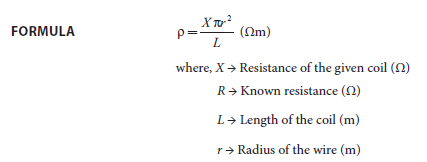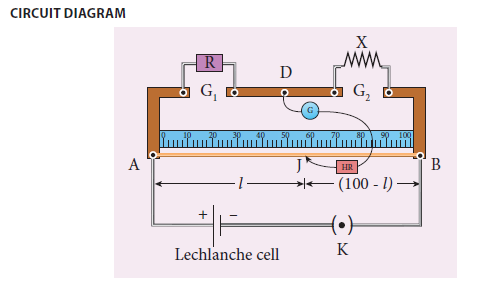PROCEDURE:

• A resistance box R is connected in the left gap and the unknown resistance X in the right gap

• A Lechlanche cell is connected across the wire of length 1 m through a key

• A sensitive galvanometer G is connected between the central strip and the jockey through a

high resistance (HR)

• With a suitable resistance included in the resistance box, the circuit is switched on

• To check the circuit connections, the jockey is pressed near one end of the wire, say A.

The galvanometer will show deflection in one direction. When the jockey is pressed near the

other end of the wire B, the galvanometer will show deflection in the opposite directions.

This ensures that the circuit connections are correct

• By moving the jockey over the wire, the point on the wire at which the galvanometer shows

null deflection i.e., balancing point J is found

• The balancing length AJ = l is noted

• The unknown resistance X1 is found using the formula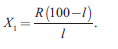• The experiment is repeated for different values of R

• The same procedure is repeated after interchanging R and X

• The unknown resistance X2 is found using the formula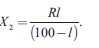• The experiment is repeated for same values of R as before

• The resistance of the given coil is found from the mean value of X1 and X2.

• The radius of the wire r is found using screw gauge

• The length of the coil L is measured using meter scale

• From the values of X, r and L, the specific resistance of the material of the wire is determined

OBSERVATION

length of the coil L = ____________________________________ cm.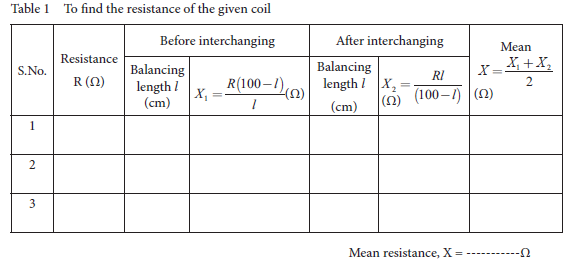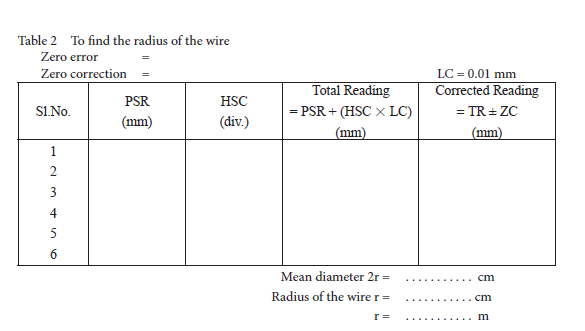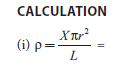RESULT

The specific resistance of the material of the given coil = _____________________(Ωm)

Note:

i) To check the circuit connections:

The meter bridge wire is touched near one end (say, end A) with jockey, galvanometer

shows a deflection in any one direction. Now the other end (say, end B) is touched. If the

galvanometer shows a deflection in the opposite direction, then the circuit connections are

correct.

ii) The usage of high resistance (HR):

The galvanometer is a very sensitive device. If any high current flows through the galvanometer,

its coil gets damaged. Therefore, in order to protect the galvanometer, a high resistance

(HR) is used. When HR is connected in series with the galvanometer, the current through it

is reduced so that the galvanometer is protected. But the balancing length is not accurate.

iii) To find the accurate balancing length:

The HR is first included in the circuit (that is, the plug key in HR is removed), the approximate

balancing length is found. Now HR is excluded in the circuit (that is, the plug key in

HR is closed), then the accurate balancing length is found.

2. HORIZONTAL COMPONENT OF EARTH’S MAGNETIC FIELD USING TANGENT GALVANOMETER

AIM: To determine the horizontal component of the Earth’s magnetic field using tangent galvanometer.

APPARATUS REQUIRED: Tangent galvanometer (TG), commutator, battery, rheostat, ammeter, key and connecting wires.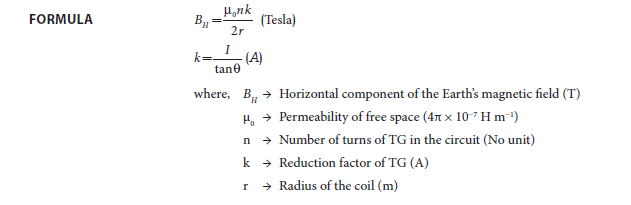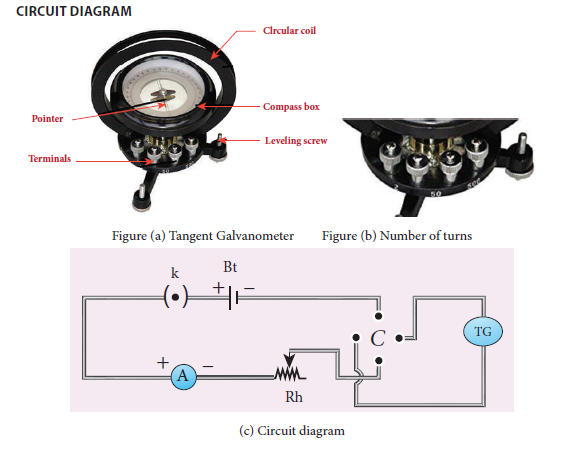PROCEDURE

• The preliminary adjustments are carried out as follows.

a. The leveling screws at the base of TG are adjusted so that the circular turntable is horizontal and the plane of the circular coil is vertical.

b. The circular coil is rotated so that its plane is in the magnetic meridian i.e., along the north south direction.

c. The compass box alone is rotated till the aluminium pointer reads 0° − 0°.

• The connections are made as shown in Figure (c).

• The number of turns n is selected and the circuit is switched on.

• The range of current through TG is chosen in such a way that the deflection of the aluminium

pointer lies between 30° − 60°.

• A suitable current is allowed to pass through the circuit, the deflections θ1 and θ2 are noted from two ends of the aluminium pointer.

• Now the direction of current is reversed using commutator C, the deflections θ3 and θ4 in the

opposite direction are noted.

• The mean value θ of θ1, θ2, θ3 and θ4 is calculated and tabulated.

• The reduction factor k is calculated for each case and it is found that k is a constant.

• The experiment is repeated for various values of current and the readings are noted and tabulated.

• The radius of the circular coil is found by measuring the circumference of the coil using a thread around the coil.

• From the values of r, n and k, the horizontal component of Earth’s magnetic field is determined.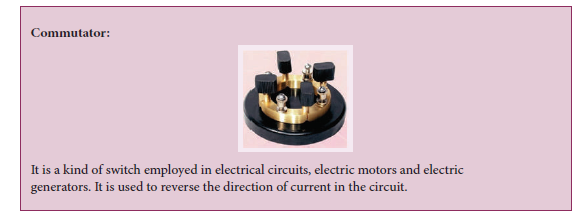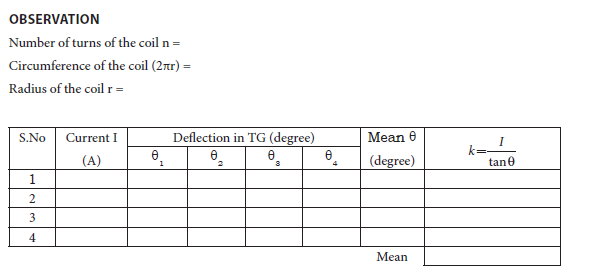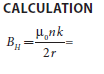RESULT

The horizontal component of Earth’s magnetic field is found to be ________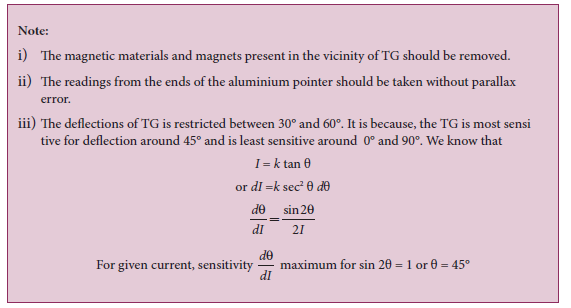3. MAGNETIC FIELD ALONG THE AXIS OF A CIRCULAR COIL – DETERMINATION OF BH

AIM: To determine the horizontal component of Earth’s magnetic field using current carrying circular coil and deflection magnetometer.

APPARATUS REQUIRED: Circular coil apparatus, compass box, rheostat, battery or power

supply, ammeter, commutator, key and connecting wires.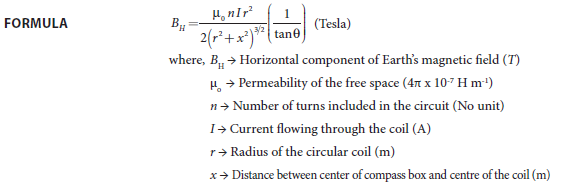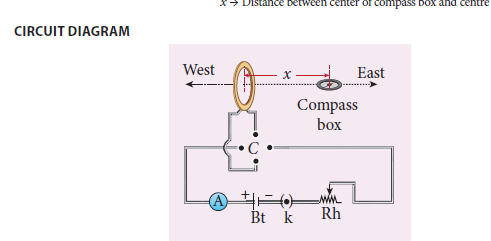PROCEDURE

• The preliminary adjustments are carried out as follows.

i. The leveling screws are adjusted so that the circular coil is vertical.

ii. The wooden bench is adjusted to be along the magnetic East-West direction i.e., along

aluminium pointer.

iii. The circular coil is rotated so that its plane is in magnetic meridian i.e., along the North-South direction.

iv. A compass box is placed with its centre coinciding with the axis of the coil.

v. The compass box alone is rotated till the aluminium pointer reads 0° − 0°

• Electrical connections are made as shown in the circuit diagram.

• The compass box is placed along its axis, with its centre at a distance x from the centre of the coil on one side

• A suitable current (1A) is passed through the coil by adjusting rheostat so that the deflection of

the aluminium pointer lies between 30o and 60o.

• The value of the current I is noted from ammeter.

• Two readings θ1 and θ2 corresponding to two ends of the pointer are noted.

• Now the direction of the current is reversed using commutator, two more readings θ3 and θ4 are noted.

• Now the compass box is taken to the other side and is kept at the same distance x.

• Four more readings θ5, θ6, θ7 and θ8 are taken as done before.

• These eight readings and their average values are tabulated.

• The experiment is repeated for another value of current, say 1.5 A by keeping the compass box at the same distance x.

• The radius of the circular coil is found by measuring the circumference of the coil using a thread around the coil.

• The number of turns n of the coil is noted.

• From the values of n, r, x and I/tanθ, the horizontal component of Earth’s magnetic field is now

found using the formula.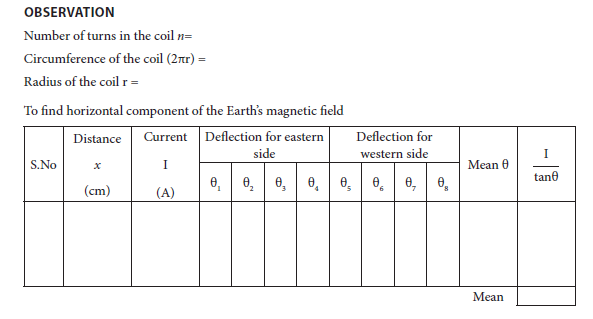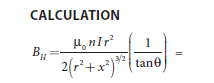RESULT

Horizontal component of the Earth’s magnetic field at a place = _________T

4. REFRACTIVE INDEX OF THE MATERIAL OF THE PRISM

AIM: To determine the refractive index of the material of a prism using spectrometer.

APPARATUS REQUIRED: Spectrometer, prism, prism clamp, sodium vapour lamp, spirit level.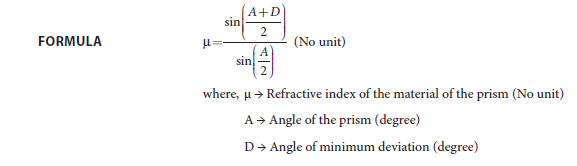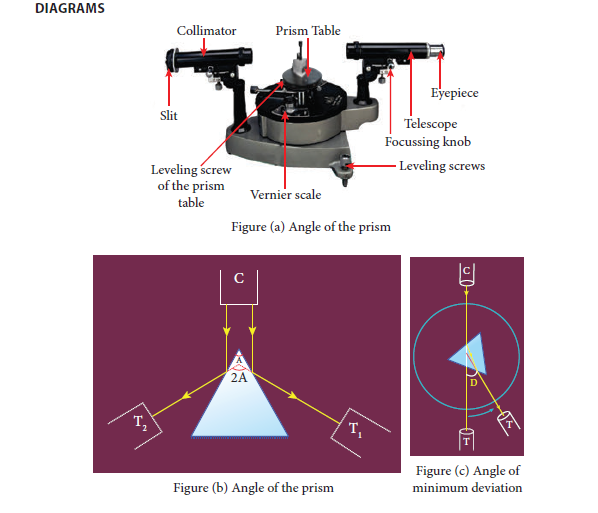PROCEDURE

1) Initial adjustments of the spectrometer

Eye-piece: The eye-piece of the telescope is adjusted so that the cross-wires are seen clearly.

Slit: The slit of the collimator is adjusted such that it is very thin and vertical.

Base of the spectrometer: The base of the spectrometer is adjusted to be horizontal using leveling screws.

Telescope: The telescope is turned towards a distant object and is adjusted till the clear inverted image of the distant object is seen. Now the telescope is adjusted to receive parallel rays.

Collimator: The telescope is brought in line with the collimator. Collimator is adjusted until a clear image of the slit is seen in the telescope. Now the collimator gives parallel rays.

Prism table: Using a spirit level, the prism table is adjusted to be horizontal with the three leveling screws provided in the prism table.

2) Determination of angle of the prism (A)

⇰The slit is illuminated by yellow light from sodium vapour lamp.

⇰The given equilateral prism is placed on the prism table in such a way that refracting edge of the prism is facing the collimator.

⇰The light emerging from the collimator is incident on both reflecting faces of the prism and is reflected.

⇰The telescope is rotated towards the left to obtain reflected image of the slit from face 1 of the prism and is fixed.

⇰Using tangential screws, the telescope is adjusted until the vertical cross-wire coincides with the reflected image of the slit.

⇰The main scale reading and vernier coincidence are noted from both vernier scales.

⇰The telescope is now rotated towards right to obtain the reflected image from face 2 of the prism. As before, the readings are taken.

⇰The difference between the two readings gives 2A from which the angle of the prism A is calculated.

3) Determination of angle of minimum deviation (D)

⇰The prism table is rotated such that the light emerging from the collimator is incident on one of the refracting faces of the prism, gets refracted and emerges out from the other refracting face.

⇰The telescope is turned to view the refracted image.

⇰Looking through the telescope, the prism table is rotated in such a direction that the image moves towards the direct ray.

⇰At one particular position, the refracted ray begins to retrace its path. The position where the refracted image returns is the position of minimum deviation.

⇰The telescope is fixed in this position and is adjusted until the vertical cross-wire coincides with the refracted image of the slit.

⇰The readings are taken from both vernier scales.

⇰The prism is now removed and the telescope is rotated to obtain the direct ray image and the readings are taken.

⇰The readings are tabulated and the difference between these two readings gives the angle of minimum deviation D.

⇰From the values of A and D, the refractive index of the material of the glass prism is determined.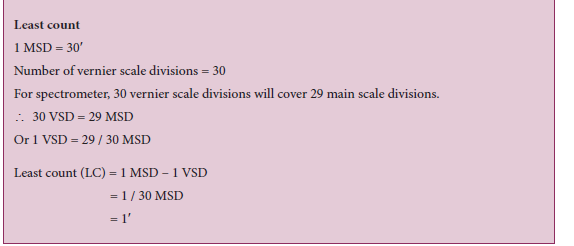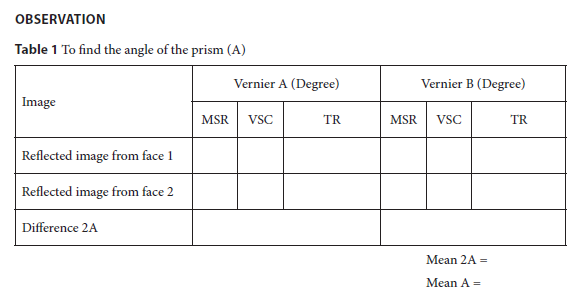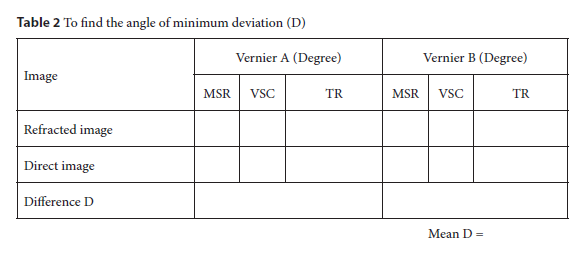RESULT

1. Angle of the Prism (A) = ………… (degree)

2. Angle of the minimum deviation of the prism (D) =…………. (degree)

3. Refractive index of the material of the Prism (μ) =…………. (No unit)

Note:

i) Once initial adjustments are done, spectrometer should not be disturbed.

ii) Total reading TR = MSR + (VSC × LC)

Where

VSC → Vernier Scale Coincidence

LC → Least count (= 1′)

5. WAVELENGTH OF THE CONSTITUENT COLOURS OF A COMPOSITE LIGHT USING DIFFRACTION GRATING AND SPECTROMETER

AIM: To find the wavelength of the constituent colours of a composite light using diffraction grating and spectrometer.

APPARATUS REQUIRED: Spectrometer, mercury vapour lamp, diffraction grating, grating table, and spirit level.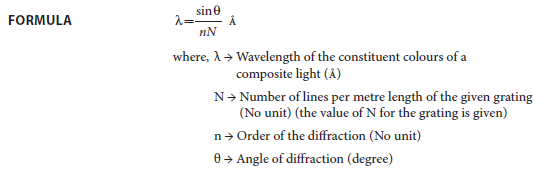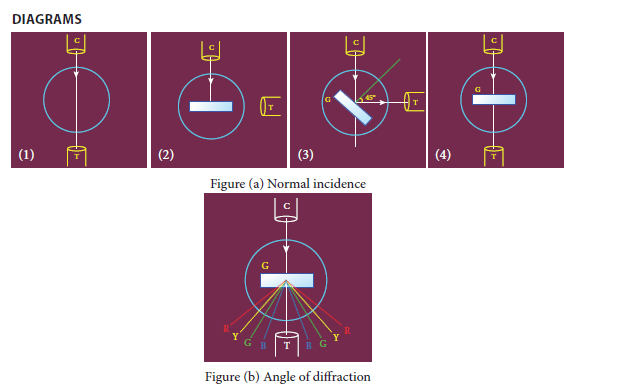PROCEDURE

1) Initial adjustments of the spectrometer

Eye-piece: The eye-piece of the telescope is adjusted so that the cross-wires are seen clearly.

Slit: The slit of the collimator is adjusted such that it is very thin and vertical.

Base of the spectrometer: The base of the spectrometer is adjusted to be horizontal using leveling screws.

Telescope: The telescope is turned towards a distant object and is adjusted till the clear image of the distant object is seen. Now the telescope is adjusted to receive parallel rays.

Collimator: The telescope is brought in line with the collimator. Collimator is adjusted until a clear image of the slit is seen in the telescope. Now the collimator gives parallel rays.

Grating table: Using a spirit level, the grating table is adjusted to be horizontal with the three leveling screws provided in the grating table.

2) Adjustment of the grating for normal incidence

⇰The slit is illuminated with a composite light (white light) from mercury vapour lamp.

⇰The telescope is brought in line with the collimator. The vertical cross-wire is made to coincide with the image of the slit (Figure (a)1).

⇰The vernier disc alone is rotated till the vernier scale reads 00 – 1800 and is fixed. This is the reading for the direct ray.

⇰The telescope is then rotated (anti-clockwise) through an angle of 900 and fixed (Figure (a)2).

⇰Now the plane transmission grating is mounted on the grating table.

⇰The grating table alone is rotated so that the light reflected from the grating coincides with vertical cross-wire of the telescope. The reflected image is white in colour (Figure (a)3).

⇰Now the vernier disc is released. The vernier disc along with grating table is rotated through an angle of 450 in the appropriate direction such that the light from the collimator is incident normally on the grating (Figure (a)4).

3) Determination of wavelength of the constituent colours of the mercury spectrum

⇰The telescope is released and is brought in line with the collimator to receive central directimage. This undispersed image is white in colour.

⇰The diffracted images of the slit are observed on either side of the direct image.

⇰The diffracted image consists of the prominent colours of mercury spectrum in increasing order of wavelength.

⇰The telescope is turned to any one side (say left) of direct image to observe first order diffracted image.

⇰The vertical cross-wire is made to coincide with the prominent spectral lines (violet, blue, yellow and red) and the readings of both vernier scales for each case are noted.

⇰Now the telescope is rotated to the right side of the direct image and the first order image is observed.

⇰The vertical cross-wire is made to coincide with the same prominent spectral lines and the readings of both vernier scales for each case are again noted.

⇰The difference between these two readings gives the value of 2θ for the particular spectral line.

⇰The number of lines per metre length of the given grating N is noted from the grating.

⇰From the values of N, n and θ, the wavelength of the prominent colours of the mercury light is determined using the given formula.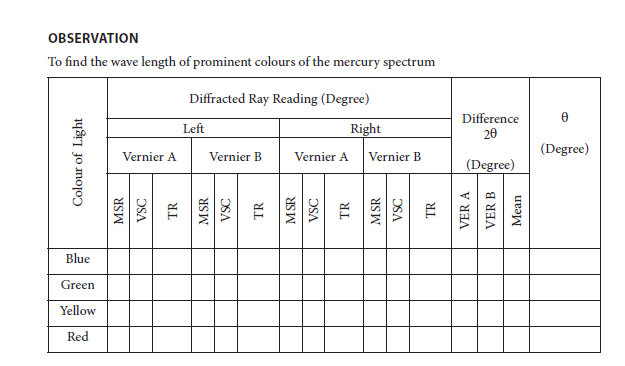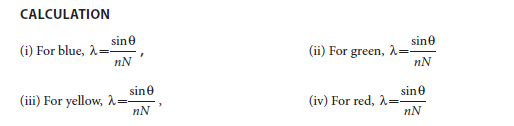RESULT

1. The wavelength of blue line = —————-m

2. The wavelength of green line = ——————m

3. The wavelength of yellow line = —————-m

4. The wavelength of red line = —————-m

Note:

i) Once initial adjustments are done, spectrometer should not be disturbed.

ii) Total reading TR = MSR + (VSC × LC)

Where

VSC → Vernier Scale Coincidence

LC → Least count (= 1′)

6. VOLTAGE-CURRENT CHARACTERISTICS OF A PN JUNCTION DIODE

AIM: To draw the voltage-current (V- I) characteristics of the PN junction

diode and to determine its knee voltage and forward resistance

APPARATUS REQUIRED: PN junction diode (IN4007), variable DC power supply,

milli-ammeter, micro-ammeter, voltmeter, resistance and connecting wires.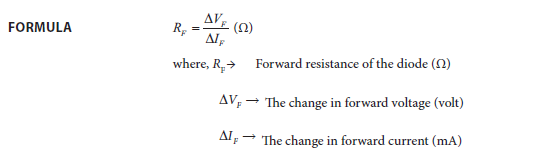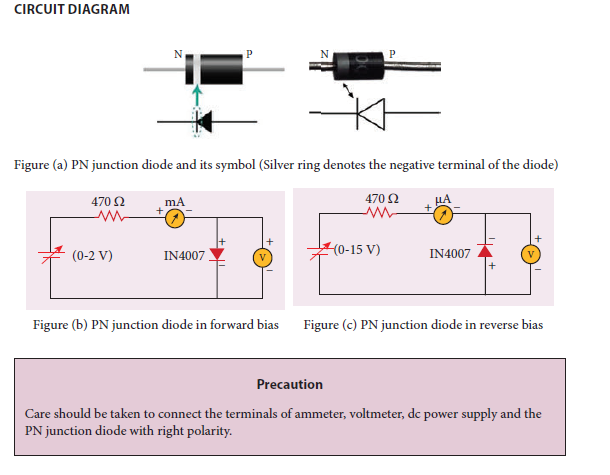PROCEDURE

i) Forward bias characteristics

• In the forward bias, the P- region of the diode is connected to the positive terminal and N-region to the negative terminal of the DC power supply.

• The connections are given as per the circuit diagram.

• The voltage across the diode can be varied with the help of the variable DC power supply.

• The forward voltage (VF) across the diode is increased from 0.1 V in steps of 0.1 V up to 0.8|V and the forward current (IF) through the diode is noted from the milli-ammeter. The readings are tabulated.

• The forward voltage VF and the forward current IF are taken as positive.

• A graph is drawn taking the forward voltage (VF) along the x-axis and the forward current (IF) along the y-axis.

• The voltage corresponding to the dotted line in the forward characteristics gives the knee voltage or threshold voltage or turn-on voltage of the diode.

• The slope in the linear portion of the forward characteristics is calculated. The reciprocal of the slope gives the forward resistance of the diode.

ii) Reverse bias characteristics

• In the reverse bias, the polarity of the DC power supply is reversed so that the P- region of the diode is connected to the negative terminal and N-region to the positive terminal of the DC power supply

• The connections are made as given in the circuit diagram.

• The voltage across the diode can be varied with the help of the variable DC power supply.

• The reverse voltage (VR) across the diode is increased from 1 V in steps of 1 V up to 5 V and the reverse current (IR) through the diode is noted from the micro-ammeter. The readings are tabulated.

• The reverse voltage VR and reverse current IR are taken as negative.

• A graph is drawn taking the reverse bias voltage (VR) along the negative x-axis and the reverse bias current (IR) along the negative y-axis.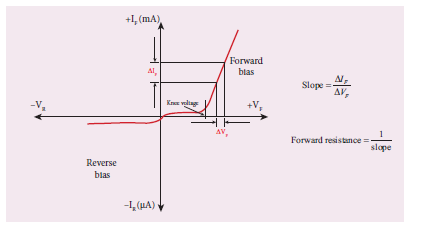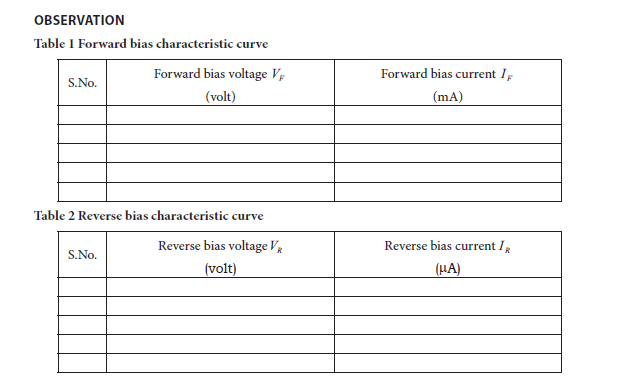CALCULATION

(i) Forward resistance RF=

(ii) knee voltage =

RESULT

The V-I characteristics of the PN junction diode are studied.

i) Knee voltage of the PN junction diode =………………V

ii) Forward resistance of the diode =………………Ω

Practical Tips

• The DC power supply voltage should be increased only up to the specified range in the forward (0 – 2V) and reverse (0 – 15V) directions. Forward bias offers very low resistance and hence an external resistance of 470Ω is connected as a safety measure.

• The voltage applied beyond this limit may damage the resistance or the diode.

• In the forward bias, the current flow will be almost zero till it crosses the junction potential or knee voltage (approximately 0.7 V). Once knee voltage is crossed, the current increases with the applied voltage.

• The diode voltage in the forward direction should be increased in steps of 0.1 V to a maximum of 0.8 V after the threshold voltage to calculate the forward resistance.

• The diode voltage in the reverse direction is increased in steps of 1 V to a maximum of 5 V. The current must be measured using micro-ammeter as the strength of current in the reverse direction is very less. This is due to the flow of the minority charge carriers called the leakage current.

7. VOLTAGE-CURRENT CHARACTERISTICS OF A ZENER DIODE

AIM: To draw the voltage-current (V-I) characteristic curves of a Zener diode and to determine its knee voltage, forward resistance and reverse breakdown voltage.

APPARATUS REQUIRED: Zener diode IZ5.6V, variable dc power supply (0 – 15V), milli ammeter, voltmeter, 470 Ω resistance, and connecting wires.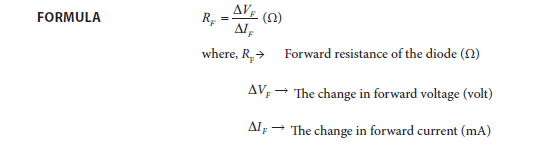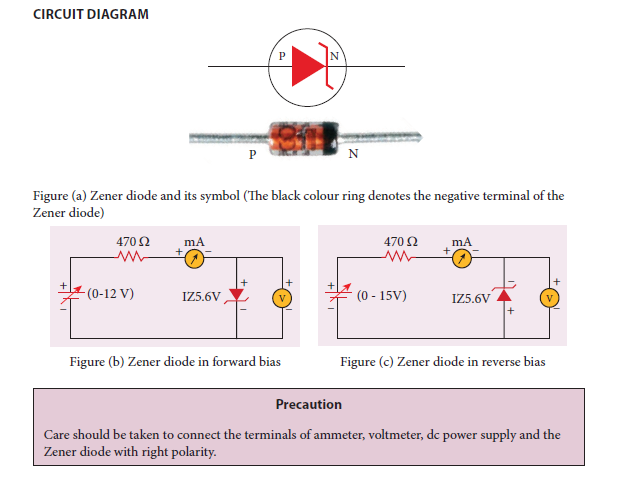PROCEDURE

i) Forward bias characteristics

• In the forward bias, the P- region of the diode is connected to the positive terminal and N-region to the negative terminal of the DC power supply.

• The connections are given as per the circuit diagram.

• The voltage across the diode can be varied with the help of the variable DC power supply.

• The forward voltage (VF) across the diode is increased from 0.1V in steps of 0.1V up to 0.8V and the forward current (IF) through the diode is noted from the milli-ammeter. The readings are tabulated.

• The forward voltage and the forward current are taken as positive.

• A graph is drawn taking the forward voltage along the x-axis and the forward current along the y-axis.

• The voltage corresponding to the dotted line in the forward characteristics gives the knee voltage or threshold voltage or turn-on voltage of the diode.

• The slope in the linear portion of the forward characteristics is calculated. The reciprocal of the slope gives the forward resistance of the diode.

ii) Reverse bias characteristics

• In the reverse bias, the polarity of the DC power supply is reversed so that the P- region of the diode is connected to the negative terminal and N-region to the positive terminal of the DC power supply

• The connections are made as given in the circuit diagram.

• The voltage across the diode can be varied with the help of the variable DC power supply.

• The reverse voltage (VR) across the diode is increased from 0.5V in steps of 0.5V up to 6V and the reverse current (IR) through the diode is noted from the milli-ammeter. The readings are tabulated.

• Initially, the voltage is increased in steps of 0.5V. When the breakdown region is approximately reached, then the input voltage may be raised in steps of, say 0.1V to find the breakdown voltage.

• The reverse voltage and reverse current are taken as negative.

• A graph is drawn taking the reverse bias voltage along negative x-axis and the reverse bias current along the negative y-axis.

• In the reverse bias, Zener breakdown occurs at a particular voltage called Zener voltage VZ(~5.6 to 5.8V) and a large amount of current flows through the diode which is the characteristics of a Zener diode.

• The breakdown voltage of the Zener diode is determined from the graph as shown.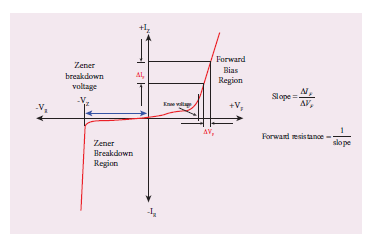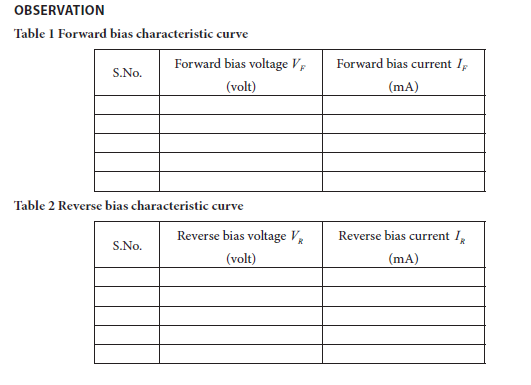CALCULATION

(i) Forward resistance RF=

(ii) knee voltage =

(iii) The breakdown voltage of the Zener diode VZ= —-V

RESULT

The V-I characteristics of the Zener diode are studied.

(i) Forward resistance RF=

(ii) knee voltage =

(iii) The breakdown voltage of the Zener diode VZ= —-V

Practical Tips

• The DC power supply voltage should be increased only up to the specified range in the

forward (0 – 2 V) and reverse (0 – 15 V) directions.

• The voltage applied beyond this limit may damage the resistor or diode.

• Zener diode functions like an ordinary PN junction diode in the forward direction.

Hence the forward characteristic is the same for both PN junction diode and Zener diode. Therefore, knee voltage and forward resistance can be determined as explained in the previous experiment.

• Unlike ordinary PN junction diode, the reverse current in Zener diode is measured using milli-ammeter due to the large flow of current.

8. CHARACTERISTICS OF A NPN-JUNCTION TRANSISTOR IN COMMON EMITTER CONFIGURATION

AIM: To study the characteristics and to determine the current gain of an NPN junction transistor in common emitter configuration.

APPARATUS REQUIRED: Transistor – BC 548/BC107, bread board, micro ammeter,

milli ammeter, voltmeters, variable DC power supply and connecting wires.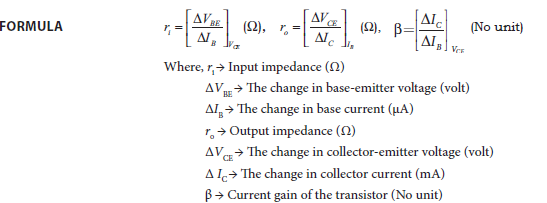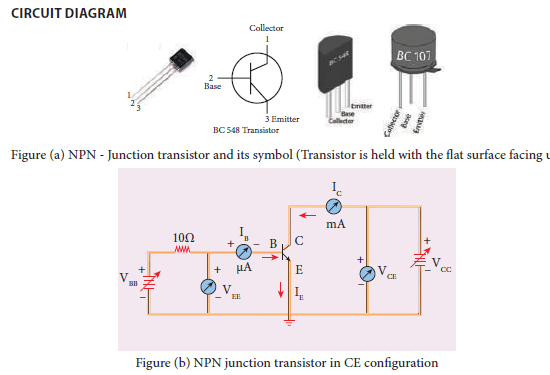Note: A resistor is connected in series with the base to prevent excess current flowing into the base.

Precautions

• Care should be taken to connect the terminals of ammeters, voltmeters, and dc power

supplies with right polarity.

• The collector and emitter terminals of the transistor must not be interchanged.

PROCEDURE

⇰The connections are given as shown in the diagram.

⇰The current and voltage at the input and output regions can be varied by adjusting the DC power supply.

(i) Input characteristic curve: VBE vs IB (VCE constant)

• The collector-emitter voltage VCE is kept constant.

• The base-emitter voltage VBE is varied in steps of 0.1V and the corresponding base current (IB) is noted. The readings are taken till VCE reaches a constant value.

• The same procedure is repeated for different values of VCE. The readings are tabulated.

• A graph is plotted by taking VBE along x-axis and IB along y-axis for both the values of VCE.

• The curves thus obtained are called the input characteristics of a transistor.

• The reciprocal of the slope of these curves gives the input impedance of the transistor.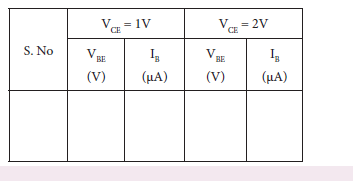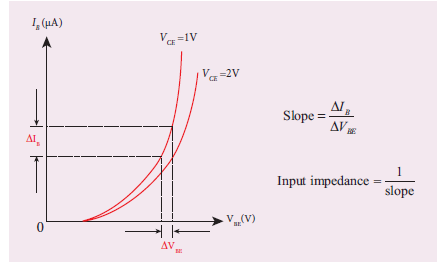(ii) Output characteristic curve: VCE vs IC (IB constant)

• The base current IB is kept constant.]

• VCE is varied in steps of 1V and the corresponding collector current IC is noted. The readings are taken until the collector current becomes almost constant.

• Initially IB is kept at 0 mA and the corresponding collector current is noted. This current is the reverse saturation current ICEO.

• The experiment is repeated for various values of IB. The readings are tabulated.

• A graph is drawn by taking VCE along x-axis and IC along y-axis for various values of IB.

• The set of curves thus obtained is called the output characteristics of a transistor.

• The reciprocal of the slope of the curve gives output impedance of the transistor.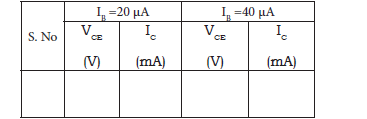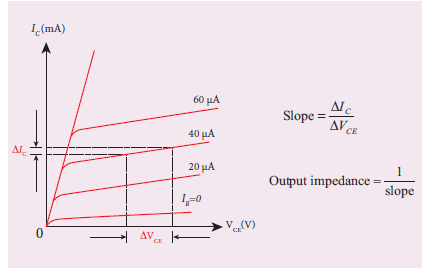(iii) Transfer characteristic curve: IB vs IC (VCE constant)

• The collector-emitter voltage VCE is kept constant.

• The base current IB is varied in steps of 10 μA and the corresponding collector current IC is noted.

• This is repeated by changing the value of VCE. The readings are tabulated.

• The transfer characteristics is a plot between the input current IB along x-axis and the output current IC along y-axis keeping VCE constant.

• The slope of the transfer characteristics plot gives the current gain β can be calculated.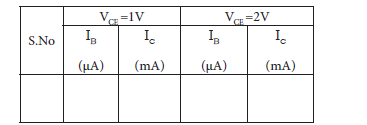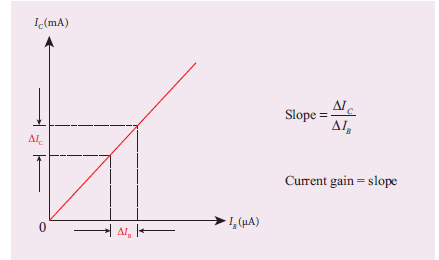RESULT

i) The input, output and transfer characteristics of the NPN junction in common emitter mode are drawn.

ii) (a) Input impedance = ________Ω

(b) Output impedance = ________Ω

(c) Current gain β = ____(no unit)

9. VERIFICATION OF TRUTH TABLES OF LOGIC GATES USING INTEGRATED CIRCUITS

AIM: To verify the truth tables of AND, OR, NOT, EX-OR, NAND and NOR gates using integrated circuits

COMPONENTS REQUIRED: AND gate (IC 7408), NOT gate (IC 7404), OR gate (IC 7432), NAND gate (IC 7400), NOR gate (IC 7402), X-OR gate (IC 7486), Power supply, Digital IC trainer kit, connecting wires.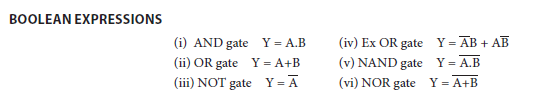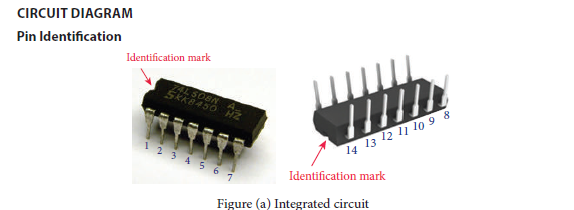Note:

The chip must be inserted in the bread board in such a way that the identification mark should be on our left side. In this position, pin numbers are counted as marked in the picture above. Pin identification is the same for all chips that are mentioned below.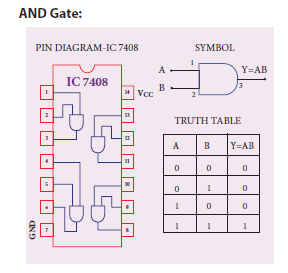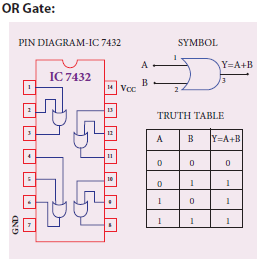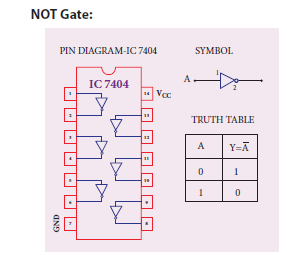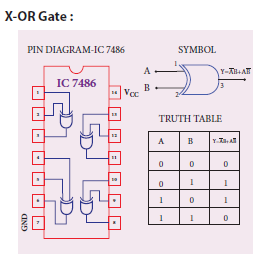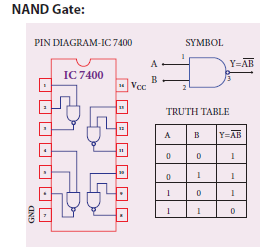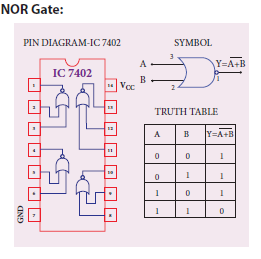PROCEDURE

• To verify the truth table of a logic gate, the suitable IC is taken and the connections are given using the circuit diagram.

• For all the ICs, 5V is applied to the pin 14 while the pin 7 is connected to the ground.

• The logical inputs of the truth table are applied and the corresponding output is noted.

• Similarly the output is noted for all other combinations of inputs.

• In this way, the truth table of a logic gate is verified.

RESULT

The truth table of logic gates AND, OR, NOT, Ex-OR, NAND and NOR using integrated circuits is verified.

Precautions

(i) VCC and ground pins must not be interchanged while making connections. Otherwise the chip will be damaged.

(ii) The pin configuration for NOR gate is different from other gates

10. VERIFICATION OF DE MORGAN’S THEOREMS

AIM: To verify De Morgan’s first and second theorems.

COMPONENTS REQUIRED: Power Supply (0 – 5V), IC 7400, 7408, 7432, 7404, and 7402, Digital IC trainer kit, connecting wires.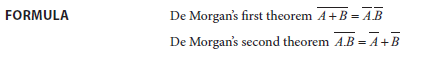CIRCUIT DIAGRAM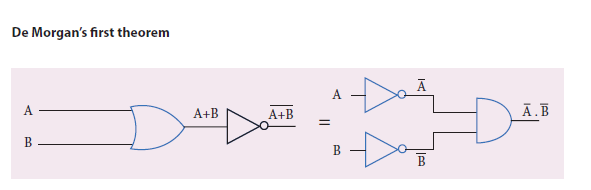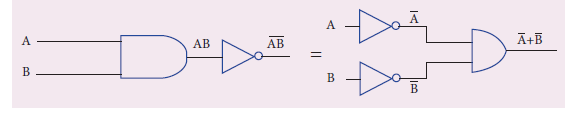PROCEDURE:

i) Verification of De Morgan’s first theorem

• The connections are made for LHS=

$$\begin{array}{l}[\overline{A+B}]\end{array}$$
of the theorem as shown in the circuit diagram using appropriate ICs.

• The output is noted and tabulated for all combinations of logical inputs of the truth table.

• The same procedure is repeated for RHS =

$$\begin{array}{l}[\overline{A}.\overline{B}]\end{array}$$
of the theorem.

• From the truth table, it can be shown that

$$\begin{array}{l}\overline{A+B}=\overline{A}.\overline{B}\end{array}$$

ii) Verification of De Morgan’s second theorem

• The connections are made for LHS

$$\begin{array}{l}[\overline{A.B}]\end{array}$$
of the theorem as shown in the

circuit diagram using appropriate ICs.

• The output is noted and tabulated for all combinations of logical inputs of the truth table.

• The same procedure is repeated for RHS

$$\begin{array}{l}[\overline{A}+\overline{B}]\end{array}$$
of the theorem.

• From the truth table, it can be shown that

$$\begin{array}{l}\overline{A+B}=\overline{A}.\overline{B}.\end{array}$$

Observation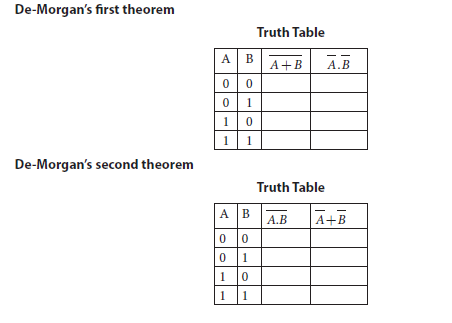RESULT

De Morgan’s first and second theorems are verified.

Note

The pin diagram for IC 7408, IC 7432 and IC 7404 can be taken from previous experiment

Precautions

VCC and ground pins must not be interchanged while making connections. Otherwise the chip will be damaged. For the ICs used, 5V is applied to the pin 14 while the pin 7 is connected to the ground.

### Tamil Nadu Board Class 12 Physics Practical – Model Questions

Other than the list of experiments, we have also compiled here some model practical questions, which the students can refer to get an idea about the type of questions they can expect in the practical exams.

SUGGESTED QUESTIONS FOR THE PRACTICAL EXAMINATION

1. Determine the resistance of a given wire using metre bridge. Also find the radius of the wire using screw gauge and hence determine the specific resistance of the material of the wire. Take at least 4 readings.

2. Determine the value of the horizontal component of the Earth’s magnetic field, using tangent galvanometer. Take at least 4 readings.

3. Determine the value of the horizontal component of the Earth’s magnetic field using the magnetic field produced along the axial line of the carrying-current circular coil. Take at least 2 readings.

4. Using the spectrometer, measure the angle of the given prism and angle of minimum deviation. Hence calculate the refractive index of the material of the prism.

5. Adjust the grating for normal incidence using the spectrometer. Determine the wavelength of green, blue, yellow and red lines of mercury spectrum (The number of lines per metre length of the grating can be noted from the grating).

6. Draw the V-I characteristics of PN junction diode and determine its forward resistance and knee voltage from forward characteristics.

7. Draw the V-I characteristics of Zener diode and determine its forward resistance and knee voltage from forward characteristics. Also find breakdown voltage of the Zener diode from reverse characteristics.

8. Draw the input and transfer characteristic curves of the given NPN junction transistor in CE mode. Find the input impedance from input characteristics and current gain from transfer characteristics.

9. Draw the output and transfer characteristic curves of the given NPN junction transistor in CE mode. Find the output impedance from output characteristics and current gain from transfer characteristics.

10. Verify the truth table of logic gates AND, NOT, Ex-OR and NOR gates using integrated circuits.

11. Verify the truth table of logic gates OR, NOT, Ex-OR and NOR gates using integrated circuits.

12. Verify De Morgan’s first and second theorems.

Students can also get more details about the TN Board and its exam resources from BYJU’S!